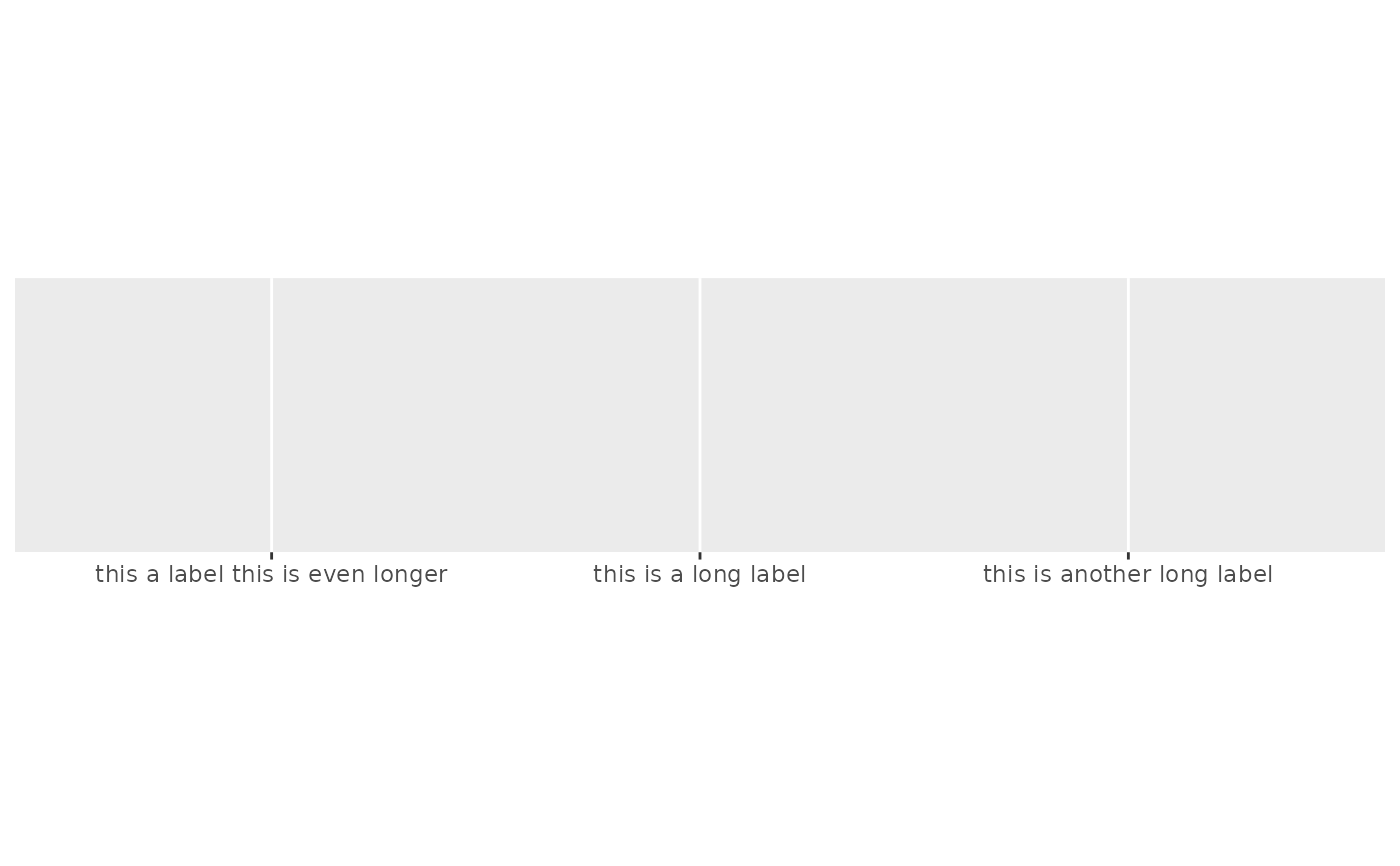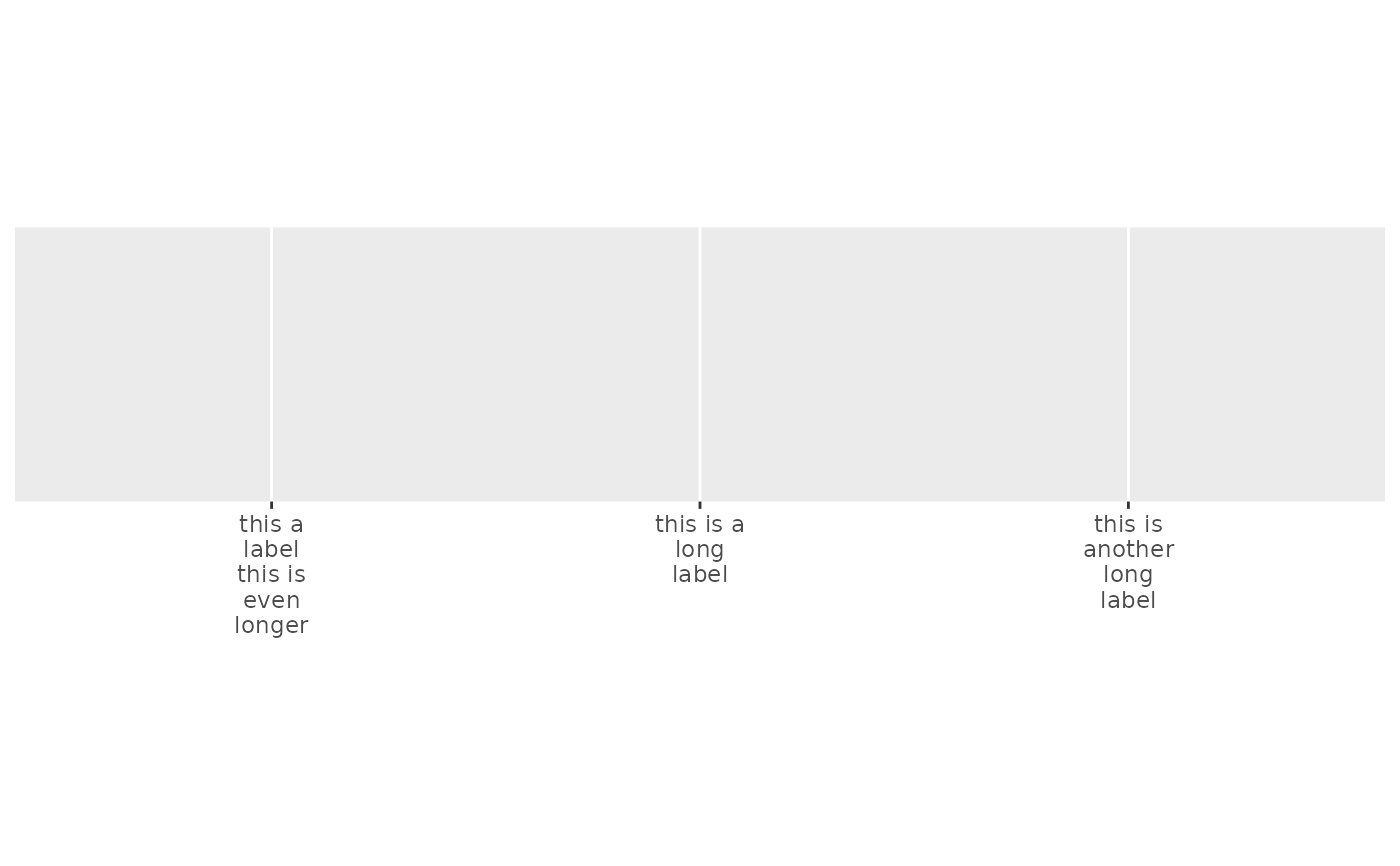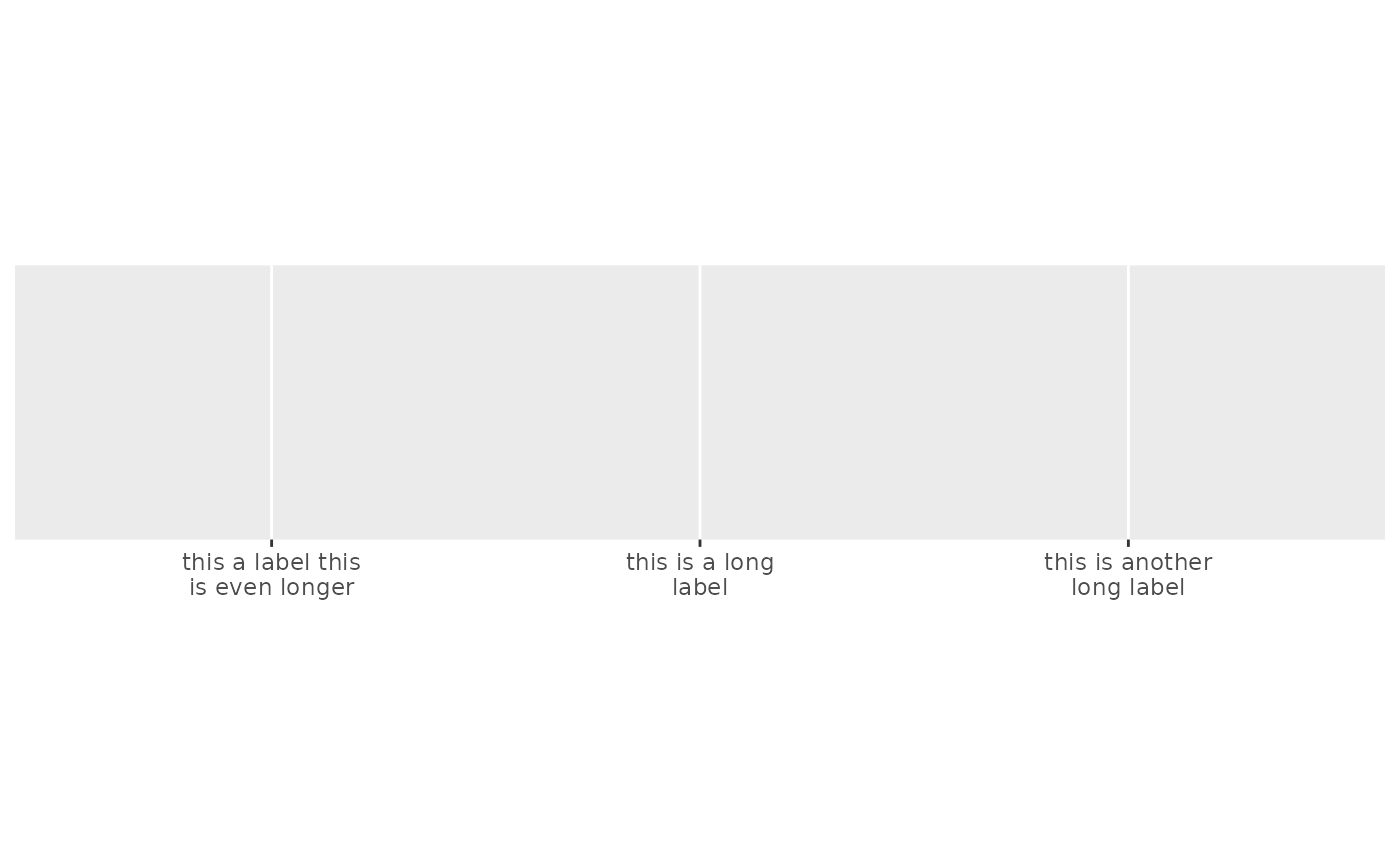Uses strwrap() to split long labels across multiple lines.

## Usage

label_wrap(width)

## Arguments

width

Number of characters per line.

## Value

All label_() functions return a "labelling" function, i.e. a function that takes a vector x and returns a character vector of length(x) giving a label for each input value.

Labelling functions are designed to be used with the labels argument of ggplot2 scales. The examples demonstrate their use with x scales, but they work similarly for all scales, including those that generate legends rather than axes.

Other labels for discrete scales: label_parse()

## Examples

x <- c(
"this is a long label",
"this is another long label",
"this a label this is even longer"
)
demo_discrete(x)
#> scale_x_discrete()demo_discrete(x, labels = label_wrap(10))
#> scale_x_discrete(labels = label_wrap(10))demo_discrete(x, labels = label_wrap(20))
#> scale_x_discrete(labels = label_wrap(20))